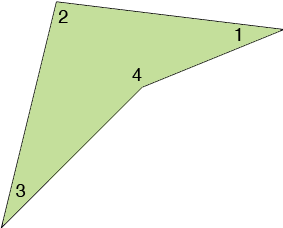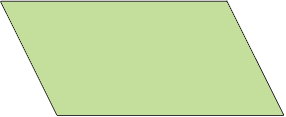SEARCH HOMEMath Central Quandaries & QueriesQuestion from xavier, a student: so, one of my math homework questions requires me to know how to find out how to find the interior angles in a parallelogram, the question is, "how many interior angles does a parallelogram have?"Hi,

Every quadrilateral has 4 interior angles.In a parallelogram the opposite angles have the same measureand hence, although there are 4 interior angles there are less than 4 different measures.

PennyMath Central is supported by the University of Regina and the Imperial Oil Foundation.